College Algebra Chapter Title (Exponential and Logarithmic Functions)

•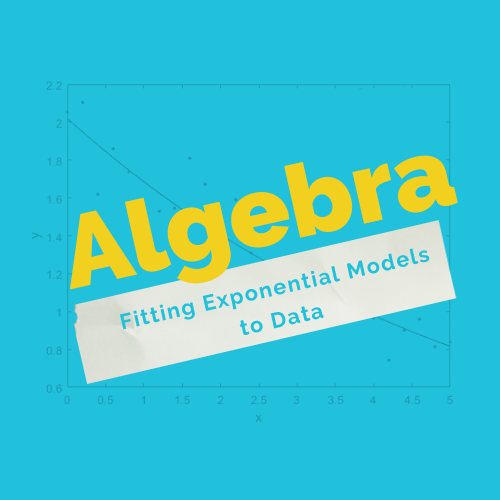Fitting Exponential Models to Data
Gallery

## Fitting Exponential Models to Data

In this course you will: Build an exponential model [...]

Fitting Exponential Models to Data2022-12-03T19:58:50+00:00
•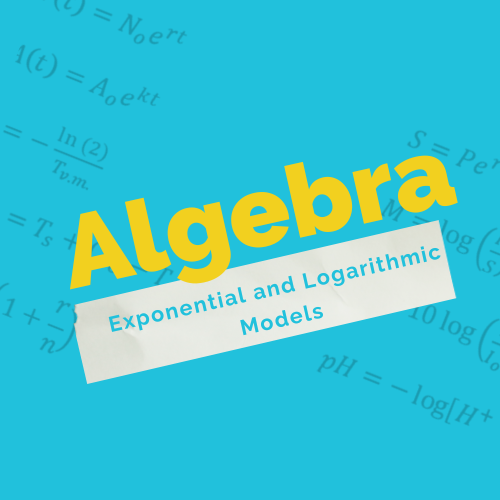Exponential and Logarithmic Models
Gallery

## Exponential and Logarithmic Models

In this course you will: Model exponential growth and [...]

Exponential and Logarithmic Models2022-12-03T20:00:13+00:00
•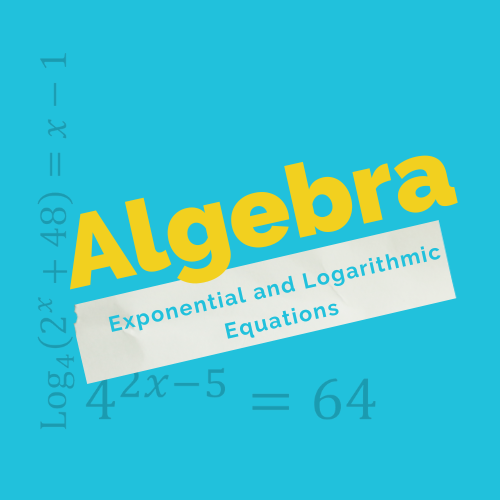Exponential and Logarithmic Equations
Gallery

## Exponential and Logarithmic Equations

In this course you will: Use like bases [...]

Exponential and Logarithmic Equations2022-12-03T20:02:19+00:00
•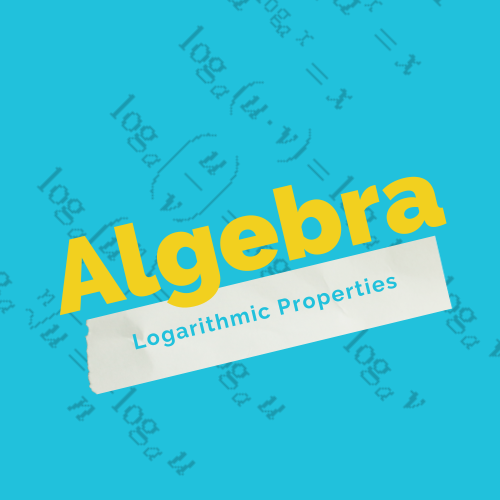Logarithmic Properties
Gallery

## Logarithmic Properties

In this course you will: Use the product rule [...]

Logarithmic Properties2022-12-03T20:03:46+00:00
•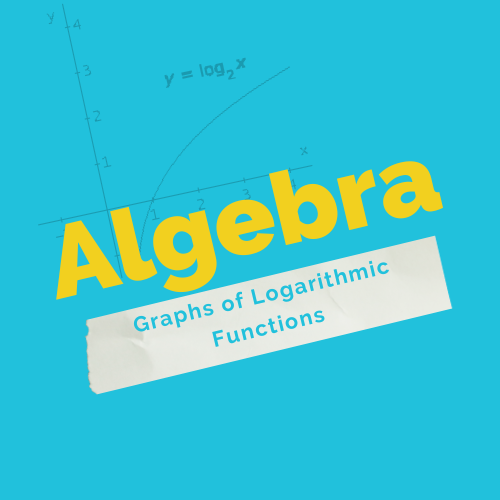Graphs of Logarithmic Functions
Gallery

## Graphs of Logarithmic Functions

In this course you will: Identify the domain [...]

Graphs of Logarithmic Functions2022-12-03T20:05:17+00:00
•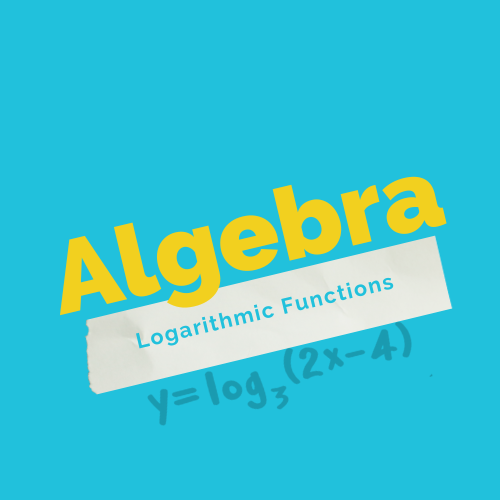Logarithmic Functions
Gallery

## Logarithmic Functions

In this course you will: Convert from logarithmic to [...]

Logarithmic Functions2022-12-03T20:07:28+00:00
•Graphs of Exponential Functions
Gallery

## Graphs of Exponential Functions

In this course you will: Graph exponential functions. Graph [...]

Graphs of Exponential Functions2022-12-03T20:09:01+00:00
•Exponential Functions
Gallery

## Exponential Functions

In this course you will: Evaluate exponential functions. Find [...]

Exponential Functions2022-12-03T20:10:41+00:00
Go to Top